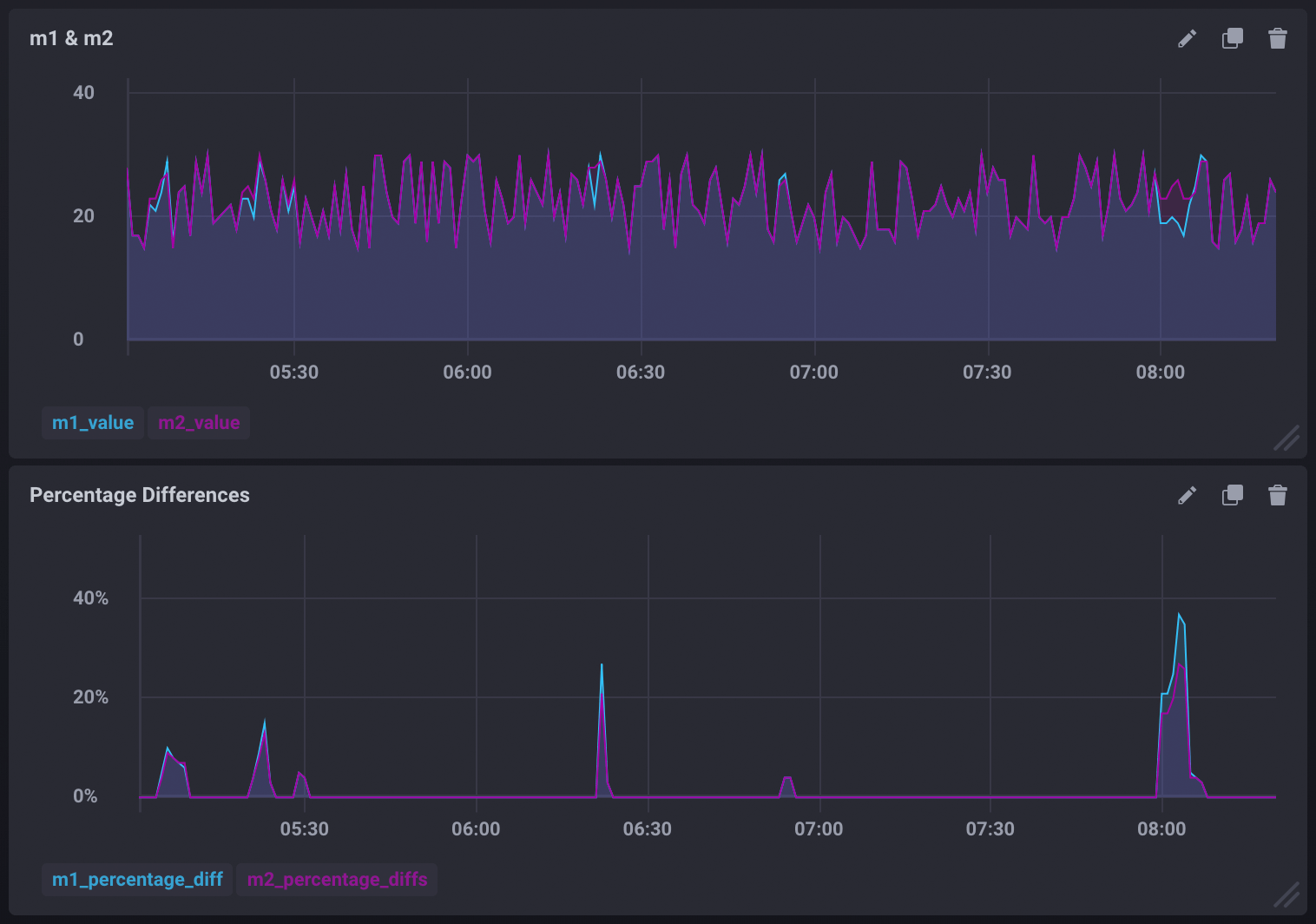# Trigger alerts by comparing two measurements

Kapacitor allows you to create alerts based on two or more measurements. In this guide, we are going to compare two measurements, `m1` and `m2`, and create an alert whenever the two measurements are different. As an added bonus, we’ll also include a query that can be used to graph the percentage difference between the two measurements.

## Comparing measurements and creating an alert

The following TICKscript streams the `m1` and `m2` measurements, joins them, compares them, and triggers an alert if the two measurements are different.

``````var window_size = 1m

// Stream m1
var m1 = stream
|from()
.measurement('m1')
|window()
.period(window_size)
.every(window_size)
.align()
|count('value')
.as('value')

// Stream m2
var m2 = stream
|from()
.measurement('m2')
|window()
.period(window_size)
.every(window_size)
.align()
|count('value')
.as('value')

// Join m1 and m2
var data = m1
|join(m2)
.as('m1', 'm2')

// Compare the joined stream and alert when m1 and m2 values are different
data
.crit(lambda: "m1.value" != "m2.value")
.message('values were not equal m1 value is {{ index .Fields "m1.value" }} m2 value is {{ index .Fields "m2.value" }}')
``````

## Graphing the percentage difference between the measurements

Use the `data` stream defined in the TICKscript above to calculate the difference between `m1` and `m2`, transform it into a float, divide that difference by the actual values of `m1` and `m2`, then multiply them by 100. This will give you the percentage difference for each. Store the difference as new fields in the `diffs` measurement:

``````data
// Calculate the difference between m1 and m2
|eval(lambda: "m1.value" - "m2.value")
.as('value_diff')
.keep()
// Calculate the % difference of m1 and m2
|eval(lambda: (float("value_diff") / float("m1.value")) * 100.0, lambda: (float("value_diff") / float("m2.value")) * 100.0)
.as('diff_percentage_m1', 'diff_percentage_m2')
// Store the calculated differences in the 'diffs' measurement
|influxDBOut()
.measurement('diffs')
.database('mydb')
.create()
``````

This can be used to create visualizations similar to:## The full TICKscript

Below is the entire, uncommented TICKscript:

``````var window_size = 1m

var m1 = stream
|from()
.measurement('m1')
|window()
.period(window_size)
.every(window_size)
.align()
|count('value')
.as('value')

var m2 = stream
|from()
.measurement('m2')
|window()
.period(window_size)
.every(window_size)
.align()
|count('value')
.as('value')

var data = m1
|join(m2)
.as('m1', 'm2')

data
.crit(lambda: "m1.value" != "m2.value")
.message('values were not equal m1 value is {{ index .Fields "m1.value" }} m2 value is {{ index .Fields "m2.value" }}')

data
|eval(lambda: "m1.value" - "m2.value")
.as('value_diff')
.keep()
|eval(lambda: (float("value_diff") / float("m1.value")) * 100.0, lambda: (float("value_diff") / float("m2.value")) * 100.0)
.as('diff_percentage_m1', 'diff_percentage_m2')
|influxDBOut()
.measurement('diffs')
.database('mydb')
.create()
``````

### Introducing InfluxDB Clustered

A highly available InfluxDB 3.0 cluster on your own infrastructure.

InfluxDB Clustered is a highly available InfluxDB 3.0 cluster built for high write and query workloads on your own infrastructure.

InfluxDB Clustered is currently in limited availability and is only available to a limited group of InfluxData customers. If interested in being part of the limited access group, please contact the InfluxData Sales team.

### The future of Flux

Flux is going into maintenance mode. You can continue using it as you currently are without any changes to your code.

Flux is going into maintenance mode and will not be supported in InfluxDB 3.0. This was a decision based on the broad demand for SQL and the continued growth and adoption of InfluxQL. We are continuing to support Flux for users in 1.x and 2.x so you can continue using it with no changes to your code. If you are interested in transitioning to InfluxDB 3.0 and want to future-proof your code, we suggest using InfluxQL.

For information about the future of Flux, see the following:

### State of the InfluxDB Cloud Serverless documentation

InfluxDB Cloud Serverless documentation is a work in progress.

The new documentation for InfluxDB Cloud Serverless is a work in progress. We are adding new information and content almost daily. Thank you for your patience!

If there is specific information you’re looking for, please submit a documentation issue.